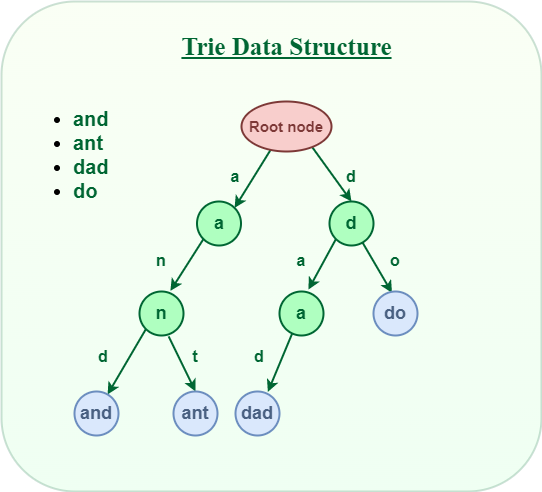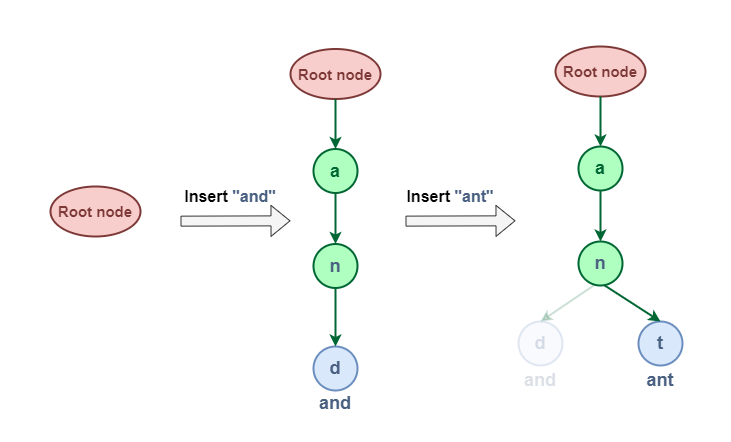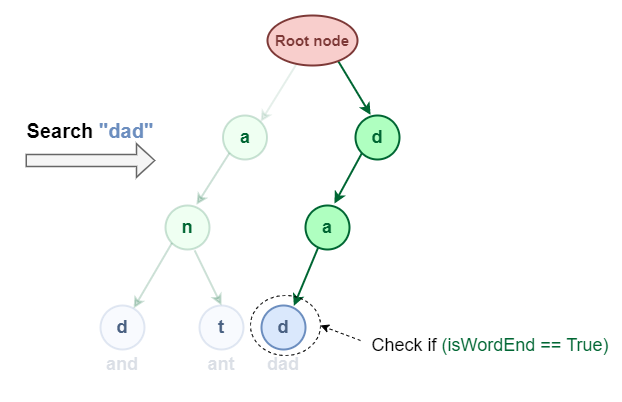Open In App

# Trie | (Insert and Search)

## What is Trie?

Trie is a type of k-ary search tree used for storing and searching a specific key from a set. Using Trie, search complexities can be brought to optimal limit (key length).

Definition: A trie (derived from retrieval) is a multiway tree data structure used for storing strings over an alphabet. It is used to store a large amount of strings. The pattern matching can be done efficiently using tries.

The trie shows words like allot, alone, ant, and, are, bat, bad. The idea is that all strings sharing common prefix should come from a common node. The tries are used in spell checking programs.

• Preprocessing pattern improves the performance of pattern matching algorithm. But if a text is very large then it is better to preprocess text instead of pattern for efficient search.
• A trie is a data structure that supports pattern matching queries in time proportional to the pattern size.

If we store keys in a binary search tree, a well balanced BST will need time proportional to M * log N, where M is the maximum string length and N is the number of keys in the tree. Using Trie, the key can be searched in O(M) time. However, the penalty is on Trie storage requirements (Please refer to Applications of Trie for more details).

Trie is also known as digital tree or prefix tree. Refer to this article for more detailed information.Trie data structure

## Structure of Trie node:

Every node of Trie consists of multiple branches. Each branch represents a possible character of keys. Mark the last node of every key as the end of the word node. A Trie node field isEndOfWord is used to distinguish the node as the end of the word node.

A simple structure to represent nodes of the English alphabet can be as follows.

## C++

 `// Trie node ``struct` `TrieNode ``{ `` ``    ``struct` `TrieNode *children[ALPHABET_SIZE];`` ``    ``// isEndOfWord is true if the node `` ``    ``// represents end of a word `` ``    ``bool` `isEndOfWord; ``}; `

## Java

 `// Trie node ``class` `TrieNode``{``    ``TrieNode[] children = ``new` `TrieNode[ALPHABET_SIZE];``    ``// isEndOfWord is true if the node ``    ``// represents end of a word ``    ``boolean` `isEndOfWord;``}`

## Python3

 `# Python3 code` `class` `TrieNode:``    ` `    ``# Trie node class``    ``def` `__init__(``self``):``        ``self``.children ``=` `[``None` `for` `_ ``in` `range``(``26``)]` `        ``# isEndOfWord is True if node represent the end of the word``        ``self``.isEndOfWord ``=` `False`

## C#

 `// Trie node``class` `TrieNode``{``    ``TrieNode[] children = ``new` `TrieNode[ALPHABET_SIZE];``    ``// isEndOfWord is true if the node``    ``// represents end of a word``    ``bool` `isEndOfWord;``}`

## Javascript

 `// TrieNode class in JS``class TrieNode``{``    ``constructor()``    ``{``        ``this``.children = ``new` `Array(26)``        ` `        ``// isEndOfWord is True if node represent the end of the word``        ``self.isEndOfWord = ``false``;``        ` `    ``}``}` `// This code is contributed by phasing17.`

## Insert Operation in Trie:

Inserting a key into Trie is a simple approach.

• Every character of the input key is inserted as an individual Trie node. Note that the children is an array of pointers (or references) to next-level trie nodes.
• The key character acts as an index to the array children
• If the input key is new or an extension of the existing key, construct non-existing nodes of the key, and mark the end of the word for the last node.
• If the input key is a prefix of the existing key in Trie, Simply mark the last node of the key as the end of a word.

The key length determines Trie depth.

The following picture explains the construction of trie using keys given in the example below.Insertion operation

1. In tries the keys are searched using common prefixes. Hence it is faster. The lookup of keys depends upon the height in case of binary search tree.

2. Tries take less space when they contain a large number of short strings. As nodes are shared between the keys.

3. Tries help with longest prefix matching, when we want to find the key.

### Comparison of tries with hash table

1. Looking up data in a trie is faster in worst case as compared to imperfect hash table.

2. There are no collisions of different keys in a trie.

3. In trie if single key is associated with more than one value then it resembles buckets in hash table.

4. There is no hash function in trie.

5. Sometimes data retrieval from tries is very much slower than hashing.

6. Representation of keys a string is complex. For example, representing floating point numbers using strings is really complicated in tries.

7. Tries always take more space than hash tables.

8. Tries are not available in programming tool it. Hence implementation of tries has to be done from scratch.

### Applications of tries

1. Tries has an ability to insert, delete or search for the entries. Hence they are used in building dictionaries such as entries for telephone numbers, English words.

2. Tries are also used in spell-checking softwares.

## Search Operation in Trie:

Searching for a key is similar to the insert operation. However, It only compares the characters and moves down. The search can terminate due to the end of a string or lack of key in the trie.

• In the former case, if the isEndofWord field of the last node is true, then the key exists in the trie.
• In the second case, the search terminates without examining all the characters of the key, since the key is not present in the trie.Searching in Trie

Note: Insert and search costs O(key_length), however, the memory requirements of Trie is O(ALPHABET_SIZE * key_length * N) where N is the number of keys in Trie. There are efficient representations of trie nodes (e.g. compressed trie, ternary search tree, etc.) to minimize the memory requirements of the trie.

## How to implement a Trie Data Structure?

• Create a root node with the help of TrieNode() constructor.
• Store a collection of strings that have to be inserted in the trie in a vector of strings say, arr.
• Inserting all strings in Trie with the help of the insert() function,
• Search strings with the help of search() function.

Below is the implementation of the above approach:

## C++

 `// C++ implementation of search and insert``// operations on Trie``#include ``using` `namespace` `std;` `const` `int` `ALPHABET_SIZE = 26;` `// trie node``struct` `TrieNode``{``    ``struct` `TrieNode *children[ALPHABET_SIZE];` `    ``// isEndOfWord is true if the node represents``    ``// end of a word``    ``bool` `isEndOfWord;``};` `// Returns new trie node (initialized to NULLs)``struct` `TrieNode *getNode(``void``)``{``    ``struct` `TrieNode *pNode =  ``new` `TrieNode;` `    ``pNode->isEndOfWord = ``false``;` `    ``for` `(``int` `i = 0; i < ALPHABET_SIZE; i++)``        ``pNode->children[i] = NULL;` `    ``return` `pNode;``}` `// If not present, inserts key into trie``// If the key is prefix of trie node, just``// marks leaf node``void` `insert(``struct` `TrieNode *root, string key)``{``    ``struct` `TrieNode *pCrawl = root;` `    ``for` `(``int` `i = 0; i < key.length(); i++)``    ``{``        ``int` `index = key[i] - ``'a'``;``        ``if` `(!pCrawl->children[index])``            ``pCrawl->children[index] = getNode();` `        ``pCrawl = pCrawl->children[index];``    ``}` `    ``// mark last node as leaf``    ``pCrawl->isEndOfWord = ``true``;``}` `// Returns true if key presents in trie, else``// false``bool` `search(``struct` `TrieNode *root, string key)``{``    ``struct` `TrieNode *pCrawl = root;` `    ``for` `(``int` `i = 0; i < key.length(); i++)``    ``{``        ``int` `index = key[i] - ``'a'``;``        ``if` `(!pCrawl->children[index])``            ``return` `false``;` `        ``pCrawl = pCrawl->children[index];``    ``}` `    ``return` `(pCrawl->isEndOfWord);``}` `// Driver``int` `main()``{``    ``// Input keys (use only 'a' through 'z'``    ``// and lower case)``    ``string keys[] = {``"the"``, ``"a"``, ``"there"``,``                    ``"answer"``, ``"any"``, ``"by"``,``                     ``"bye"``, ``"their"` `};``    ``int` `n = ``sizeof``(keys)/``sizeof``(keys);` `    ``struct` `TrieNode *root = getNode();` `    ``// Construct trie``    ``for` `(``int` `i = 0; i < n; i++)``        ``insert(root, keys[i]);` `    ``// Search for different keys``    ``char` `output[] = {``"Not present in trie"``, ``"Present in trie"``};` `    ``// Search for different keys``    ``cout<<``"the"``<<``" --- "``<

## C

 `// C implementation of search and insert operations``// on Trie``#include ``#include ``#include ``#include ` `#define ARRAY_SIZE(a) sizeof(a)/sizeof(a)` `// Alphabet size (# of symbols)``#define ALPHABET_SIZE (26)` `// Converts key current character into index``// use only 'a' through 'z' and lower case``#define CHAR_TO_INDEX(c) ((int)c - (int)'a')` `// trie node``struct` `TrieNode``{``    ``struct` `TrieNode *children[ALPHABET_SIZE];` `    ``// isEndOfWord is true if the node represents``    ``// end of a word``    ``bool` `isEndOfWord;``};` `// Returns new trie node (initialized to NULLs)``struct` `TrieNode *getNode(``void``)``{``    ``struct` `TrieNode *pNode = NULL;` `    ``pNode = (``struct` `TrieNode *)``malloc``(``sizeof``(``struct` `TrieNode));` `    ``if` `(pNode)``    ``{``        ``int` `i;` `        ``pNode->isEndOfWord = ``false``;` `        ``for` `(i = 0; i < ALPHABET_SIZE; i++)``            ``pNode->children[i] = NULL;``    ``}` `    ``return` `pNode;``}` `// If not present, inserts key into trie``// If the key is prefix of trie node, just marks leaf node``void` `insert(``struct` `TrieNode *root, ``const` `char` `*key)``{``    ``int` `level;``    ``int` `length = ``strlen``(key);``    ``int` `index;` `    ``struct` `TrieNode *pCrawl = root;` `    ``for` `(level = 0; level < length; level++)``    ``{``        ``index = CHAR_TO_INDEX(key[level]);``        ``if` `(!pCrawl->children[index])``            ``pCrawl->children[index] = getNode();` `        ``pCrawl = pCrawl->children[index];``    ``}` `    ``// mark last node as leaf``    ``pCrawl->isEndOfWord = ``true``;``}` `// Returns true if key presents in trie, else false``bool` `search(``struct` `TrieNode *root, ``const` `char` `*key)``{``    ``int` `level;``    ``int` `length = ``strlen``(key);``    ``int` `index;``    ``struct` `TrieNode *pCrawl = root;` `    ``for` `(level = 0; level < length; level++)``    ``{``        ``index = CHAR_TO_INDEX(key[level]);` `        ``if` `(!pCrawl->children[index])``            ``return` `false``;` `        ``pCrawl = pCrawl->children[index];``    ``}` `    ``return` `(pCrawl->isEndOfWord);``}` `// Driver``int` `main()``{``    ``// Input keys (use only 'a' through 'z' and lower case)``    ``char` `keys[] = {``"the"``, ``"a"``, ``"there"``, ``"answer"``, ``"any"``,``                     ``"by"``, ``"bye"``, ``"their"``};` `    ``char` `output[] = {``"Not present in trie"``, ``"Present in trie"``};`  `    ``struct` `TrieNode *root = getNode();` `    ``// Construct trie``    ``int` `i;``    ``for` `(i = 0; i < ARRAY_SIZE(keys); i++)``        ``insert(root, keys[i]);` `    ``// Search for different keys``    ``printf``(``"%s --- %s\n"``, ``"the"``, output[search(root, ``"the"``)] );``    ``printf``(``"%s --- %s\n"``, ``"these"``, output[search(root, ``"these"``)] );``    ``printf``(``"%s --- %s\n"``, ``"their"``, output[search(root, ``"their"``)] );``    ``printf``(``"%s --- %s\n"``, ``"thaw"``, output[search(root, ``"thaw"``)] );` `    ``return` `0;``}`

## Java

 `// Java implementation of search and insert operations``// on Trie``public` `class` `Trie {``    ` `    ``// Alphabet size (# of symbols)``    ``static` `final` `int` `ALPHABET_SIZE = ``26``;``    ` `    ``// trie node``    ``static` `class` `TrieNode``    ``{``        ``TrieNode[] children = ``new` `TrieNode[ALPHABET_SIZE];``     ` `        ``// isEndOfWord is true if the node represents``        ``// end of a word``        ``boolean` `isEndOfWord;``        ` `        ``TrieNode(){``            ``isEndOfWord = ``false``;``            ``for` `(``int` `i = ``0``; i < ALPHABET_SIZE; i++)``                ``children[i] = ``null``;``        ``}``    ``};``     ` `    ``static` `TrieNode root;``    ` `    ``// If not present, inserts key into trie``    ``// If the key is prefix of trie node,``    ``// just marks leaf node``    ``static` `void` `insert(String key)``    ``{``        ``int` `level;``        ``int` `length = key.length();``        ``int` `index;``     ` `        ``TrieNode pCrawl = root;``     ` `        ``for` `(level = ``0``; level < length; level++)``        ``{``            ``index = key.charAt(level) - ``'a'``;``            ``if` `(pCrawl.children[index] == ``null``)``                ``pCrawl.children[index] = ``new` `TrieNode();``     ` `            ``pCrawl = pCrawl.children[index];``        ``}``     ` `        ``// mark last node as leaf``        ``pCrawl.isEndOfWord = ``true``;``    ``}``     ` `    ``// Returns true if key presents in trie, else false``    ``static` `boolean` `search(String key)``    ``{``        ``int` `level;``        ``int` `length = key.length();``        ``int` `index;``        ``TrieNode pCrawl = root;``     ` `        ``for` `(level = ``0``; level < length; level++)``        ``{``            ``index = key.charAt(level) - ``'a'``;``     ` `            ``if` `(pCrawl.children[index] == ``null``)``                ``return` `false``;``     ` `            ``pCrawl = pCrawl.children[index];``        ``}``     ` `        ``return` `(pCrawl.isEndOfWord);``    ``}``     ` `    ``// Driver``    ``public` `static` `void` `main(String args[])``    ``{``        ``// Input keys (use only 'a' through 'z' and lower case)``        ``String keys[] = {``"the"``, ``"a"``, ``"there"``, ``"answer"``, ``"any"``,``                         ``"by"``, ``"bye"``, ``"their"``};``     ` `        ``String output[] = {``"Not present in trie"``, ``"Present in trie"``};``     ` `     ` `        ``root = ``new` `TrieNode();``     ` `        ``// Construct trie``        ``int` `i;``        ``for` `(i = ``0``; i < keys.length ; i++)``            ``insert(keys[i]);``     ` `        ``// Search for different keys``        ``if``(search(``"the"``) == ``true``)``            ``System.out.println(``"the --- "` `+ output[``1``]);``        ``else` `System.out.println(``"the --- "` `+ output[``0``]);``        ` `        ``if``(search(``"these"``) == ``true``)``            ``System.out.println(``"these --- "` `+ output[``1``]);``        ``else` `System.out.println(``"these --- "` `+ output[``0``]);``        ` `        ``if``(search(``"their"``) == ``true``)``            ``System.out.println(``"their --- "` `+ output[``1``]);``        ``else` `System.out.println(``"their --- "` `+ output[``0``]);``        ` `        ``if``(search(``"thaw"``) == ``true``)``            ``System.out.println(``"thaw --- "` `+ output[``1``]);``        ``else` `System.out.println(``"thaw --- "` `+ output[``0``]);``       ` `    ``}``}``// This code is contributed by Sumit Ghosh`

## Python3

 `# Python program for insert and search``# operation in a Trie` `class` `TrieNode:``    ` `    ``# Trie node class``    ``def` `__init__(``self``):``        ``self``.children ``=` `[``None``]``*``26` `        ``# isEndOfWord is True if node represent the end of the word``        ``self``.isEndOfWord ``=` `False` `class` `Trie:``    ` `    ``# Trie data structure class``    ``def` `__init__(``self``):``        ``self``.root ``=` `self``.getNode()` `    ``def` `getNode(``self``):``    ` `        ``# Returns new trie node (initialized to NULLs)``        ``return` `TrieNode()` `    ``def` `_charToIndex(``self``,ch):``        ` `        ``# private helper function``        ``# Converts key current character into index``        ``# use only 'a' through 'z' and lower case``        ` `        ``return` `ord``(ch)``-``ord``(``'a'``)`  `    ``def` `insert(``self``,key):``        ` `        ``# If not present, inserts key into trie``        ``# If the key is prefix of trie node,``        ``# just marks leaf node``        ``pCrawl ``=` `self``.root``        ``length ``=` `len``(key)``        ``for` `level ``in` `range``(length):``            ``index ``=` `self``._charToIndex(key[level])` `            ``# if current character is not present``            ``if` `not` `pCrawl.children[index]:``                ``pCrawl.children[index] ``=` `self``.getNode()``            ``pCrawl ``=` `pCrawl.children[index]` `        ``# mark last node as leaf``        ``pCrawl.isEndOfWord ``=` `True` `    ``def` `search(``self``, key):``        ` `        ``# Search key in the trie``        ``# Returns true if key presents``        ``# in trie, else false``        ``pCrawl ``=` `self``.root``        ``length ``=` `len``(key)``        ``for` `level ``in` `range``(length):``            ``index ``=` `self``._charToIndex(key[level])``            ``if` `not` `pCrawl.children[index]:``                ``return` `False``            ``pCrawl ``=` `pCrawl.children[index]` `        ``return` `pCrawl.isEndOfWord` `# driver function``def` `main():` `    ``# Input keys (use only 'a' through 'z' and lower case)``    ``keys ``=` `[``"the"``,``"a"``,``"there"``,``"anaswe"``,``"any"``,``            ``"by"``,``"their"``]``    ``output ``=` `[``"Not present in trie"``,``              ``"Present in trie"``]` `    ``# Trie object``    ``t ``=` `Trie()` `    ``# Construct trie``    ``for` `key ``in` `keys:``        ``t.insert(key)` `    ``# Search for different keys``    ``print``(``"{} ---- {}"``.``format``(``"the"``,output[t.search(``"the"``)]))``    ``print``(``"{} ---- {}"``.``format``(``"these"``,output[t.search(``"these"``)]))``    ``print``(``"{} ---- {}"``.``format``(``"their"``,output[t.search(``"their"``)]))``    ``print``(``"{} ---- {}"``.``format``(``"thaw"``,output[t.search(``"thaw"``)]))` `if` `__name__ ``=``=` `'__main__'``:``    ``main()` `# This code is contributed by Atul Kumar (www.facebook.com/atul.kr.007)`

## C#

 `// C# implementation of search and``// insert operations on Trie``using` `System;``    ` `public` `class` `Trie``{``    ` `    ``// Alphabet size (# of symbols)``    ``static` `readonly` `int` `ALPHABET_SIZE = 26;``    ` `    ``// trie node``    ``class` `TrieNode``    ``{``        ``public` `TrieNode[] children = ``new` `TrieNode[ALPHABET_SIZE];``    ` `        ``// isEndOfWord is true if the node represents``        ``// end of a word``        ``public` `bool` `isEndOfWord;``        ` `        ``public` `TrieNode()``        ``{``            ``isEndOfWord = ``false``;``            ``for` `(``int` `i = 0; i < ALPHABET_SIZE; i++)``                ``children[i] = ``null``;``        ``}``    ``};``    ` `    ``static` `TrieNode root;``    ` `    ``// If not present, inserts key into trie``    ``// If the key is prefix of trie node,``    ``// just marks leaf node``    ``static` `void` `insert(String key)``    ``{``        ``int` `level;``        ``int` `length = key.Length;``        ``int` `index;``    ` `        ``TrieNode pCrawl = root;``    ` `        ``for` `(level = 0; level < length; level++)``        ``{``            ``index = key[level] - ``'a'``;``            ``if` `(pCrawl.children[index] == ``null``)``                ``pCrawl.children[index] = ``new` `TrieNode();``    ` `            ``pCrawl = pCrawl.children[index];``        ``}``    ` `        ``// mark last node as leaf``        ``pCrawl.isEndOfWord = ``true``;``    ``}``    ` `    ``// Returns true if key``    ``// presents in trie, else false``    ``static` `bool` `search(String key)``    ``{``        ``int` `level;``        ``int` `length = key.Length;``        ``int` `index;``        ``TrieNode pCrawl = root;``    ` `        ``for` `(level = 0; level < length; level++)``        ``{``            ``index = key[level] - ``'a'``;``    ` `            ``if` `(pCrawl.children[index] == ``null``)``                ``return` `false``;``    ` `            ``pCrawl = pCrawl.children[index];``        ``}``    ` `        ``return` `(pCrawl.isEndOfWord);``    ``}``    ` `    ``// Driver``    ``public` `static` `void` `Main()``    ``{``        ``// Input keys (use only 'a'``        ``// through 'z' and lower case)``        ``String []keys = {``"the"``, ``"a"``, ``"there"``, ``"answer"``,``                        ``"any"``, ``"by"``, ``"bye"``, ``"their"``};``    ` `        ``String []output = {``"Not present in trie"``, ``"Present in trie"``};``    ` `    ` `        ``root = ``new` `TrieNode();``    ` `        ``// Construct trie``        ``int` `i;``        ``for` `(i = 0; i < keys.Length ; i++)``            ``insert(keys[i]);``    ` `        ``// Search for different keys``        ``if``(search(``"the"``) == ``true``)``            ``Console.WriteLine(``"the --- "` `+ output);``        ``else` `Console.WriteLine(``"the --- "` `+ output);``        ` `        ``if``(search(``"these"``) == ``true``)``            ``Console.WriteLine(``"these --- "` `+ output);``        ``else` `Console.WriteLine(``"these --- "` `+ output);``        ` `        ``if``(search(``"their"``) == ``true``)``            ``Console.WriteLine(``"their --- "` `+ output);``        ``else` `Console.WriteLine(``"their --- "` `+ output);``        ` `        ``if``(search(``"thaw"``) == ``true``)``            ``Console.WriteLine(``"thaw --- "` `+ output);``        ``else` `Console.WriteLine(``"thaw --- "` `+ output);``        ` `    ``}``}` `/* This code contributed by PrinciRaj1992 */`

## PHP

 `node = ``new` `TrieNode();``    ``}``  ` `    ``// Inserts a word into the trie.` `    ``public` `function` `insert(``\$word``)``    ``{``        ``\$count` `= ``strlen``(``\$word``);``        ``\$node` `= ``\$this``->node;``        ``for` `(``\$i` `= 0; ``\$i` `< ``\$count``; ``\$i``++) {``            ``\$char` `= ``\$word``[``\$i``];``            ``if` `(``array_key_exists``(``\$char``, ``\$node``->children)) {``                ``\$node` `= ``\$node``->children[``\$char``];``                ``continue``;``            ``}``            ``\$node``->children[``\$char``] = ``new` `TrieNode();``            ``\$node` `= ``\$node``->children[``\$char``];``        ``}``        ``\$node``->isEnd = true;``    ``}``  ` `    ``// Returns if the word is in the trie.``     ` `    ``public` `function` `search(``\$word``): bool``    ``{``        ``\$count` `= ``strlen``(``\$word``);``        ``\$node` `= ``\$this``->node;``        ``for` `(``\$i` `= 0; ``\$i` `< ``\$count``; ``\$i``++) {``            ``\$char` `= ``\$word``[``\$i``];``            ``if` `(!``array_key_exists``(``\$char``, ``\$node``->children)) {``                ``return` `false;``            ``}``            ``\$node` `= ``\$node``->children[``\$char``];``        ``}` `        ``return` `\$node``->isEnd;``    ``}``}``    ``\$keys` `= ``array``(``"the"``,``"a"``,``"there"``,``"answer"``,``"any"``,``"by"``,``"their"``);` `    ``# Trie object``    ``\$t` `= ``new` `Trie();` `    ``# Constructing trie``    ``foreach` `(``\$keys` `as` `\$key``) {``        ``\$t``->insert(``\$key``);``    ``}``  ` `   ``# Searching different words``   ``print` `(``\$t``->search(``"the"``)==1)?(``"the ---- Present in trie\n"``)``       ``🙁``"the ---- Not present in trie\n"``);``   ``print` `(``\$t``->search(``"this"``)==1)?(``"this ---- Present in trie\n"``)``       ``🙁``"this ---- Not present in trie\n"``);``   ``print` `(``\$t``->search(``"they"``)==1)?(``"they ---- Present in trie\n"``)``       ``🙁``"they ---- Not present in trie\n"``);``   ``print` `(``\$t``->search(``"thaw"``)==1)?(``"thaw ---- Present in trie"``)``       ``🙁``"thaw ---- Not present in trie"``);` `# This code is contributed by Sajal Aggarwal.`  `?>`

## Javascript

 ``

Output

```the --- Present in trie
these --- Not present in trie
their --- Present in trie
thaw --- Not present in trie```

## Complexity Analysis of Trie Data Structure:

Related Articles:

Practice Problems:

Recent Articles on Trie